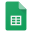# CAMP Special Lecture

### CAMP Special Lecture

This series of lectures is free and open to everyone.

If you would join this, in order to let us know, please register here before June 14.

• Title. Alternating Permutations

• Lecturer. Richard P. Stanley, MIT, USA

• Date. June 29 (Mon.) - July 3 (Fri.), 2015

• Time. 14:00 (June 29-30, July 1, July 3), 15:30 (July 2)

• Venue. CAMP & NIMS

### Detailed Time & Venue.

• NIMS Seminar Room (3rd floor), 2:00 PM, June 29, 2015

• NIMS Seminar Room (3rd floor), 2:00 PM, June 30, 2015

• CAMP Seminar Room (M), 2:00 PM, July 1, 2015

• CAMP Seminar Room (L), 3:30 PM, July 2, 2015

• CAMP Seminar Room (L), 2:00 PM, July 3, 2015

### Abstract. (for all lectures)

A permutation a1 a2 ... an of 1, 2, ..., n is alternating if a1 > a2 < a3 > a4 < ... . Alternating permutations have many fascinating properties related to combinatorics, geometry, representation theory, probability theory, and other areas. We will survey the highlights of this subject.

Lecture 1. (June 29, 14:00) Euler numbers and André's theorem

The number of alternating permutations of 1, 2, ..., n is denoted En and is called an Euler number. The first theorem on alternating permutations is the generating function

n≥0 En xn / n! = tan(x) + sec(x)

due to D. André in 1879. Some other combinatorial objects are also counted by the Euler numbers.

Lecture 2. (June 30, 14:00) Convex polytopes and alternating permutations

There are two interesting convex polytopes whose volume is En / n!. One of them is given by xi ≥ 0 (1 ≤ i ≤ n) and xi + xi+1 ≤ 1 (1 ≤ i ≤ n-1). They fit into a more general context of order polytopes and chain polytopes of posets.

Lecture 3. (July 1, 14:00) Longest alternating subsequences

What can one say about the length of the longest alternating subsequence of a random permutation? This theory is analogous but simpler than the better-known theory of the longest increasing subsequence of a random permutation. For example, if n>1 then the expected length of the longest alternating subsequence of a random permutation of 1, 2, ..., n is exactly (4n+1)/6.

Lecture 4. (July 2, 15:30) A symmetric group character related to alternating permutations

H. O. Foulkes defined a certain character χ of the symmetric group Sn whose values χ(w) are either 0 or ±Ek for some 1 ≤ k ≤ n (depending on w). This character allows us to enumerate certain classes of alternating permutations using representation theory. For instance, if p is prime then the number of alternating permutations in Sp that are also p-cycles is equal to

(Ep - (-1)(p-1)/2) / p.

No elementary proof is known.

Lecture 5. (July 3, 14:00) The cd-index of the symmetric group

The cd-index of Sn is a noncommutative polynomial in the indeterminates c and d that encodes a lot of combinatorial information. The number of terms in this polynomial is En, and there are interesting connections with alternating permutations.

### ParticipantsParticipants